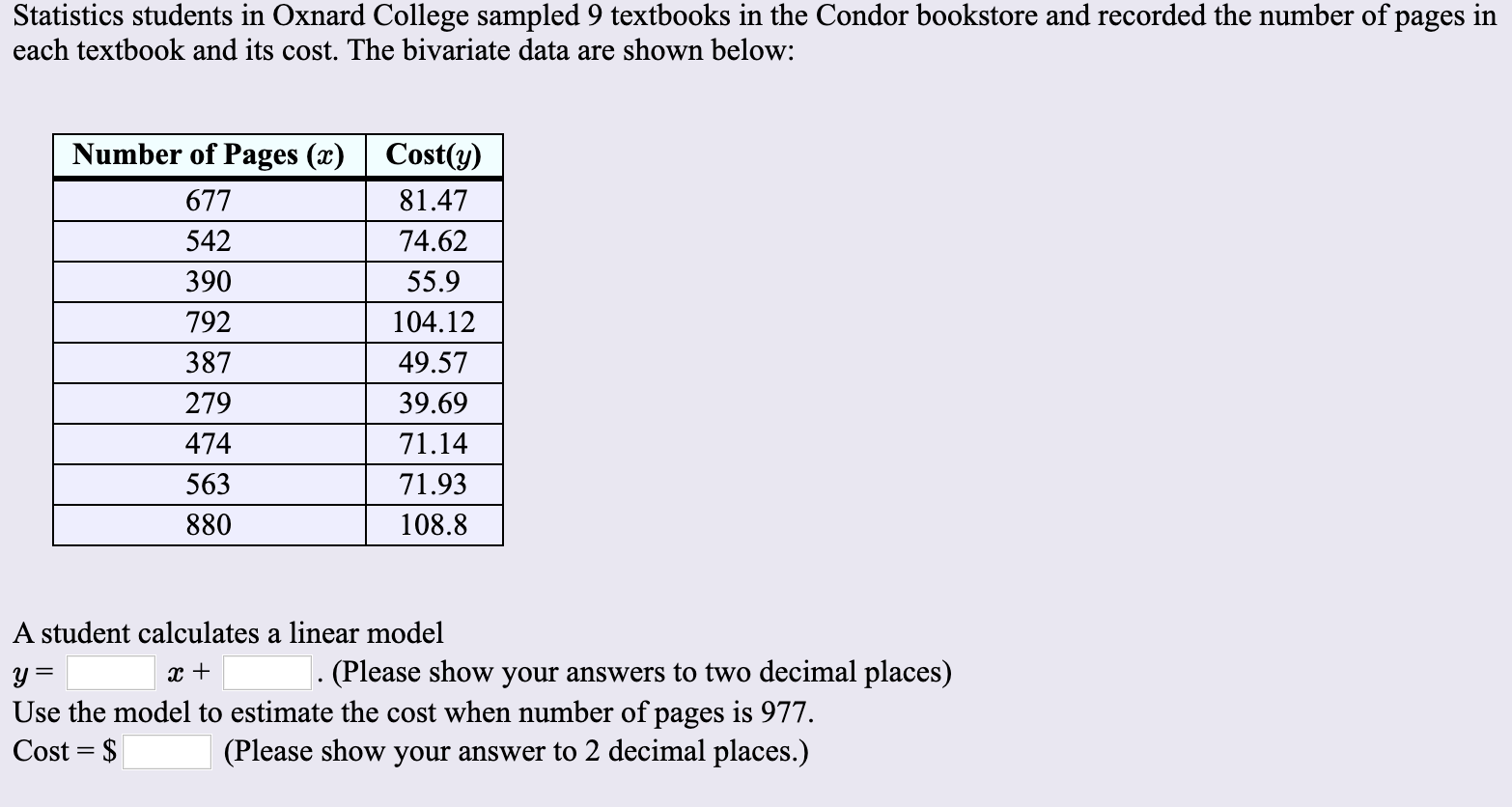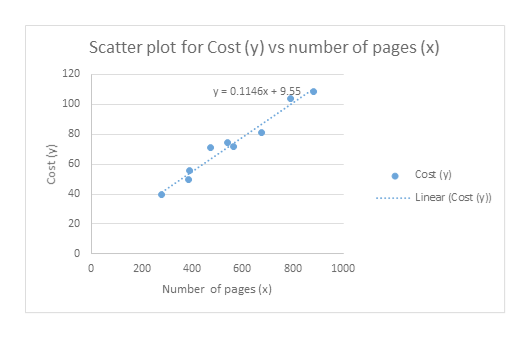# Statistics students in Oxnard College sampled 9 textbooks in the Condor bookstore and recorded the number of pages ineach textbook and its cost. The bivariate data are shown below:Number of Pages (x)Cost(y)67781.4754274.6239055.9792104.1238749.5727939.6971.1447456371.93880108.8A student calculates a linear model. (Please show your answers to two decimal places)Use the model to estimate the cost when number of pages is 977Cost \$(Please show your answer to 2 decimal places.)

Questionhelp_outlineImage TranscriptioncloseStatistics students in Oxnard College sampled 9 textbooks in the Condor bookstore and recorded the number of pages in each textbook and its cost. The bivariate data are shown below: Number of Pages (x) Cost(y) 677 81.47 542 74.62 390 55.9 792 104.12 387 49.57 279 39.69 71.14 474 563 71.93 880 108.8 A student calculates a linear model . (Please show your answers to two decimal places) Use the model to estimate the cost when number of pages is 977 Cost \$ (Please show your answer to 2 decimal places.) fullscreen
check_circle

Step 1

The linear model obtained using scatter diagram is given below:

The line that best fits the data is least-squares regression line.

The procedure to draw scatterplot using EXCEL is as follows.

• Select the data Number of Pages (x) and Cost(y).
• Go to Insert.
• Select Scatter under Charts.
• Right click on the obtained chart, check linear, Display Equation on chart.

The scatter diagram for the data that is obtained using EXCEL is as follows:help_outlineImage TranscriptioncloseScatter plot for Cost (y) vs number of pages (x) 120 y 0.1146x+9.55 100 60 Cost (y) 40 Linear (Cost (y) 20 0 200 400 600 800 1000 Number of pages (x) 80 (A) as0 fullscreen
Step 2

From the scatter diagram, the linear model i...

### Want to see the full answer?

See Solution

#### Want to see this answer and more?

Solutions are written by subject experts who are available 24/7. Questions are typically answered within 1 hour.*

See Solution
*Response times may vary by subject and question.
Tagged in

### Statistics